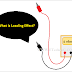In simple words, when the load affects the power source then it is called the Loading Effect. We know that a practical power source always has an internal resistance or impedance. When we connect a load with that power source the load impedance will affect the source impedance, usually, it reduces the supply voltage. In normal electrical circuits, the loading effect does not affect more or it can be negligible. But in the case of electrical measurement, it must be noted to get the accuracy for the measurement. So the loading effect in measurement is a very important factor and it cannot be neglected.

In an electrical measurement, the loading effect not only affects the power source even it also affects the whole system. If we understand it with an example, it will be clear to us. So let's understand with an example. For example, a low-sensitivity voltameter is connected in parallel with the power source and load. Generally, the resistance of a good voltameter should be very high. But in case, if a low-sensitivity voltmeter that has low resistance is connected in parallel with the system, it will lower the overall resistance of the circuit, so an error will occur.

Loading effect also occurs when a low effective impedance load circuit is connected to a power source that has high comparatively high impedance. This happens because the low load impedance lowers the overall circuit impedance so a high current will flow in the circuit.

We know that a good ammeter has a very low internal resistance. The internal resistance indicates its sensitivity. If the internal resistance of the ammeter is very low then the sensitivity will be very high but if the internal resistance is high the sensitivity will be low. So when a low sensitivity ammeter(that has high internal resistance) is connected in series between the power source and load, the overall resistance of the circuit will be increased which will lower the flow of current. So the ammeter cannot be able to measure the actual current flow or current consumption by the load.

For example, if we connect a 50ohm ammeter in series with a 100ohm circuit. The overall resistance of the circuit will be 150 ohm which is 3 times greater than the internal resistance of the ammeter. So, definitely, it will impact the measurement of the current and voltage.

A good voltmeter is generally designed with high internal resistance. If the internal resistance of the voltmeter is very then the sensitivity also be very high but if the internal resistance is low then the sensitivity also be low. So if a low-sensitivity voltmeter that has low internal resistance is connected in parallel with a circuit it will pass a very high current through it so the actual voltage of the circuit will be decreased. The voltameter will be not able to measure or show the actual voltage of the circuit.

What is Loading Effect in Measurement? ExamplesReviewed by Author on January 19, 2022 Rating: 5